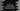# C program to check if a number is even or odd using bitwise operator# C program to Check if a number is even or odd using bitwise operator

In this tutorial, we will learn how to find if a number is odd or even using Bitwise operator. We know that if a number is divisible by 2, it is an even number and if not it is called an odd number. We can easily divide the number by 2 and check if it is odd or even using a modulus, but in this tutorial, we will learn how to use one bitwise operator to find out the same.

## Explanation :

Bitwise AND operator is used to find out if a number is even or odd. This operator is denoted by &. We will do AND operation of the number with 1. Based on this result, we can say the number is even or odd. Let’s try it with few numbers : 8 :

``````8   => 1 0 0 0
1   => 0 0 0 1
-------------
8&1 => 0 0 0 0``````

9 :

``````9   => 1 0 0 1
1   => 0 0 0 1
-------------
9&1 => 0 0 0 1``````

22 :

``````22    => 1 0 1 1 0
1     => 0 0 0 0 1
-----------------
22&1  => 0 0 0 0 0``````

111 :

``````111   => 1 1 0 1 1 1 1
1     => 0 0 0 0 0 0 1
----------------------
111&1 => 0 0 0 0 0 0 1``````

36 :

``````36   => 1 0 0 1 0 0
1    => 0 0 0 0 0 1
-------------------
36&1 => 0 0 0 0 0 0``````

1 :

``````1   => 0 0 0 1
1   => 0 0 0 1
---------------
1&1 => 0 0 0 1``````

From the above examples, we have seen that : For 8, if we AND it with 1, the result is 0. Same result for 22 and 36. But for 9,111,1 the result is 1. So if we perform AND with a number and 1, the result is 1 if the number is odd, and 0 if the number is even.

Now, let’s try to implement it in C.

### C program :

``````#include <stdio.h>

int main()
{
int number;
printf("Enter a number to check: ");
scanf("%d", &number);

if (number & 1)
printf("%d is odd.\n", number);
else
printf("%d is even.\n", number);
return 0;
}``````

Few sample outputs of the above program :

``````Enter a number to check: 32
32 is even.

Enter a number to check: 111
111 is odd.

Enter a number to check: 10
10 is even.

Enter a number to check: 45
45 is odd.

Enter a number to check: 29
29 is odd.``````

### Conclusion :

In this tutorial, we have learned how to find out if a number is even or odd easily in C using Bitwise operator. This process is useful to quickly check a number instead of using the modulo operator. Try to run the program and drop one comment below if you have any queries.

You might also like :